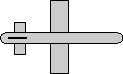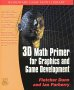# Maths - Conversion Euler To Matrix

## Prerequisites

Definition of terms:

## Equations

This depends on what conventions are used for the Euler Angles. See this page for an explanation of the conventions and standards used on this site.

[R] =
 ch*ca -ch*sa*cb + sh*sb ch*sa*sb + sh*cb sa ca*cb -ca*sb -sh*ca sh*sa*cb + ch*sb -sh*sa*sb + ch*cb

where:

• sa = sin(attitude)
• ca = cos(attitude)
• sb = sin(bank)
• cb = cos(bank)

## code

Java code to do conversion:

```/** this conversion uses NASA standard aeroplane conventions as described on page:
*   https://www.euclideanspace.com/maths/geometry/rotations/euler/index.htm
*   Coordinate System: right hand
*   Positive angle: right hand
*   Order of euler angles: heading first, then attitude, then bank
*   matrix row column ordering:
*   [m00 m01 m02]
*   [m10 m11 m12]
*   [m20 m21 m22]*/
public final void rotate(double heading, double attitude, double bank) {    // Assuming the angles are in radians.    double ch = Math.cos(heading);    double sh = Math.sin(heading);    double ca = Math.cos(attitude);    double sa = Math.sin(attitude);    double cb = Math.cos(bank);    double sb = Math.sin(bank);
m00 = ch * ca;    m01 = sh*sb - ch*sa*cb;    m02 = ch*sa*sb + sh*cb;    m10 = sa;    m11 = ca*cb;    m12 = -ca*sb;    m20 = -sh*ca;    m21 = sh*sa*cb + ch*sb;    m22 = -sh*sa*sb + ch*cb;}```

## Derivation of Equations

The matricies for rotation about individual axes are shown here.

To combine these matricies we multiply them together. They must be multipied in the right order, this is explained here. From our definitions the order of applying these rotations is heading,attitude then bank (about y,z then x). The rotation applied first goes on the right hand side of the equation but since we are working in the frame of reference of the moving object the first rotation goes on the left. Applying heading then attitude gives: (Rh * Ra), then applying bank gives:

[R] = ([Rh][Ra])[Rb]

This is expanded out here. To save space cos(bank) is written as cb and so on:

 ch 0 sh 0 1 0 -sh 0 ch
 ca -sa 0 sa ca 0 0 0 1
) * bank (rotate about x)
 1 0 0 0 cb -sb 0 sb cb

first multiply first two terms (for matrix multiplication see here) Remember order of matrix multiplication is significant.

 ch*ca -ch*sa sh sa ca 0 -sh*ca sh*sa ch

then multiply by bank:

 ch*ca -ch*sa*cb + sh*sb ch*sa*sb + sh*cb sa ca*cb -ca*sb -sh*ca sh*sa*cb + ch*sb - sh*sa*sb + ch*cb

## Issues

It is much easier to convert in this direction than to convert back from the matrix to euler angles. Therefore once we convert to matricies it is best to continue to work in matricies.

If you have a different result from that shown on this page it may be that you are using different standards, I have tried to keep the standards consistant accross this site and I have tried to define the standards that I am using here. One of these standards is that the order that the euler rotations are applied is 'NASA standard aeroplane' it uses a slightly different coordinate definition from VRML (z and y axis swapped). Also when working with aeroplanes we often work in terms of the position of external objects relative to the aircraft (i.e. the inverse of its position transform as explained here). therefore to get the expression normally used with NASA aeroplane we invert all inputs (change sign of every term with odd number of sine terms) invert output (conjugate quaternion) swap z and y for inputs (swap z and y columns) swap z and y for outputs (swap z and y rows). In the case of aircraft it can make sense to work in terms of the local frame of reference of the aircraft looking out, I have a version of this page that does that here, but be careful as this will no longer be compatible with the rest of this site.

However if you have checked these things and you still have a discrepency then I have probably made an error so please let me know here.

## Example

 we take the 90 degree rotation from this:to this:As shown here the axis angle for this rotation is:

bank = 90 degrees
attitude = 0 degrees

so substituteing this in the above formula gives:

• ca=cos(attitude) = 1
• cb=cos(bank) = 0
• sa=sin(attitude) = 0
• sb=sin(bank) = 1
[R] =
 ch*ca -ch*sa*cb + sh*sb ch*sa*sb + sh*cb sa ca*cb -ca*sb -sh*ca sh*sa*cb + ch*sb -sh*sa*sb + ch*cb
[R] =
 1 0 0 0 0 -1 0 1 0

This agrees with the matix rotations here.

## Angle Calculator and Further examples

I have put a java applet here which allows the values to be entered and the converted values shown along with a graphical representation of the orientation.

Also further examples in 90 degree steps here

 metadata block see also: Correspondence about this page Book Shop - Further reading. Where I can, I have put links to Amazon for books that are relevant to the subject, click on the appropriate country flag to get more details of the book or to buy it from them.3D Math Primer - Aimed at complete beginners to vector and matrix algebra.

This site may have errors. Don't use for critical systems.